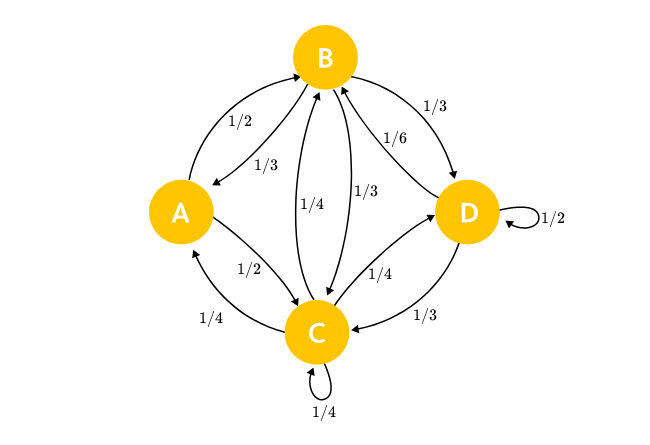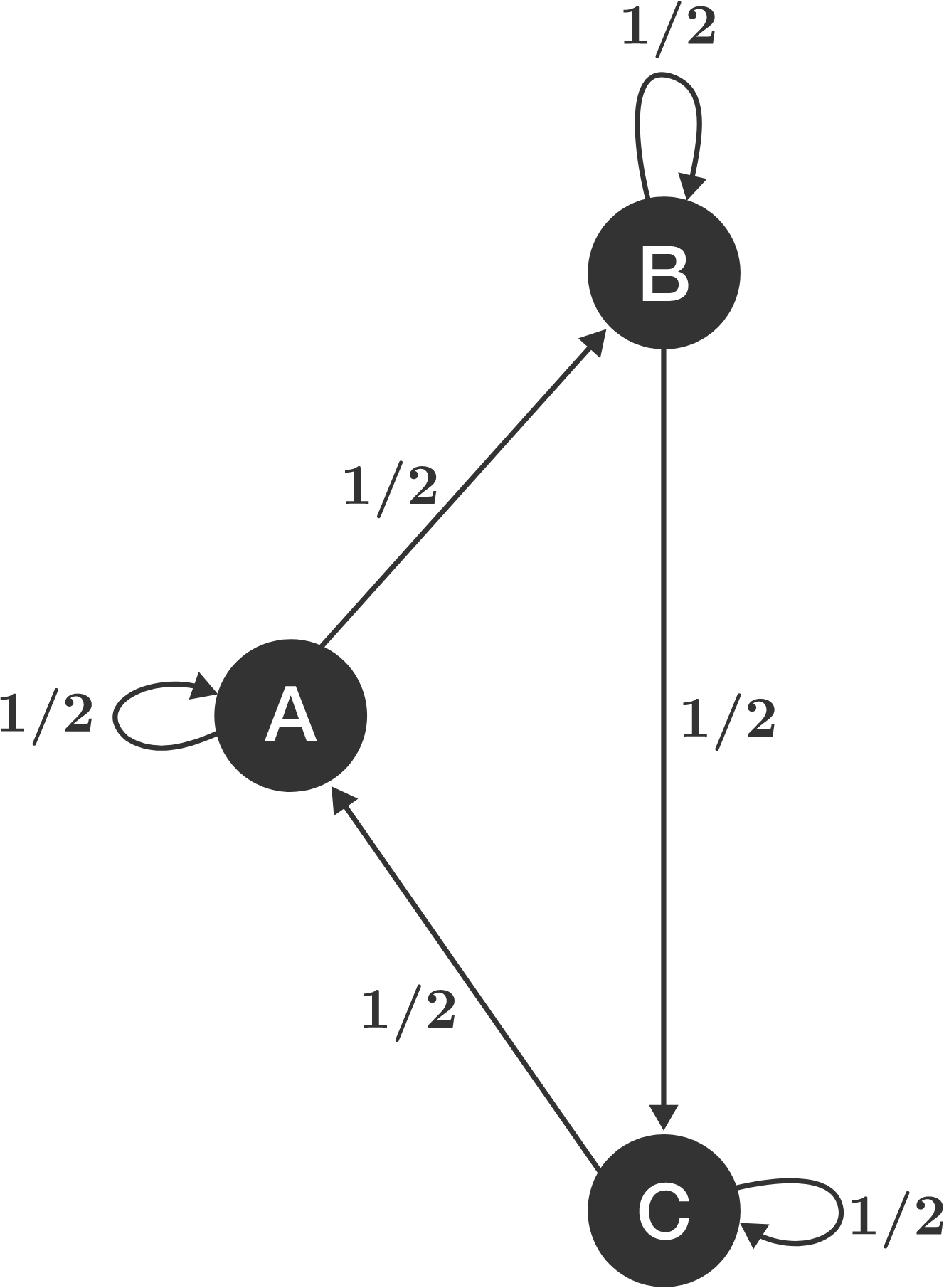Probability

# Markov Chains - Transience and Recurrence

Biologists can model a population's genotype distribution across generations with a Markov chain. Suppose there are two individuals with genotype Aa, and in each successive generation, two individuals are selected from the (numerous) offspring of the previous generation. These pairs of individuals form the possible states: AA and AA; AA and Aa; Aa and Aa; AA and aa; Aa and aa; aa and aa. Note that the first and last states listed are absorbing states. If the process reaches the state Aa and Aa, and two independent choices are made from the offspring population, determine the probability that the process reaches the state AA and AA in the very next step.

Recall that a child receives one allele (letter) from each parent, with a $50\%$ probability of either allele being selected; for instance, the parents with genotype Aa will have a child with genotype AA with probability $25\%$, Aa with probability $50\%$ and aa with probability $25\%$.

Which of the following are true?

(1) Suppose state $i$ is null recurrent. The expected number of returns to state $i$ is infinite.
(2) Suppose state $i$ is positive recurrent. The expected number of returns to state $i$ is finite.
(3) Suppose state $i$ is transient. The expected number of steps needed to return to state $i$ is not finite.

A Markov chain has states that are the natural numbers $1, \, 2, 3, \ldots$ and the transition probabilities at state $n > 1$ are probability $\tfrac{1}{n}$ to go to $n+1$, probability $\tfrac{1}{n}$ to go to $n - 1$ and probability $1 - \tfrac{2}{n}$ to remain at $n$ (state $1$ simply has probability $1$ of going to state $2$).

Are any states periodic?
Are any states transient?

If a process starts at state A, what is the expected number of steps until it reaches state D?If a process starts at state A, what is the expected number of steps until it returns to state A?×